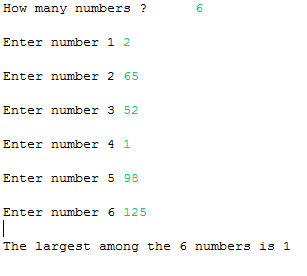## Thursday, 8 January 2015

### Java Program to find the Smallest Element in Array

This is a Java Program which finds the smallest element in an array.

Here initially first element in array and  its next element are compared array and array , if array is lesser, then the value is stored in array and now the second element is increased 2 .3 4 .. until the last element is reached.

PROGRAM :
```package codingcorner.in;

import java.util.Scanner;

public class SmallestElementArray {
public static void main(String[] args) {
int i, n;
System.out.print("How many numbers ?\t");
Scanner scan = new Scanner(System.in);
n = scan.nextInt();
int array[] = new int[n];
for (i = 0; i < n; i++) {
System.out.print("\nEnter number " + (i + 1));
array[i] = scan.nextInt();
}
scan.close();
for (i = 0; i < n; i++) {
if (array > array[i])
array = array[i];
}
System.out.print("\nThe largest among the " + n + " numbers is "
+ array);
}
}```

OUTPUT :Java - Smallest element in an array